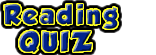This text is meant to accompany class discussions. It is not everything there is to know about energy. It is meant as a  prep for class. More detailed notes and examples are given in the class notes, presentations, and demonstrations (click here.)

Work

Work is the term used to describe the transference of energy. Work is given the math variable "W." That's a capital "W." Because it is used to describe the flow of energy it has the same units as energy, Joules.

Work is the "dot product" of two vectors. The dot product gives an answer that is a scalar. This means that work is component of force parallel to the displacement times the displacement. Mathematically this is written as,The easy application of this formulas when the force and displacement point in the same direction as show in the animation below.

But what if the force and the displacement are not parallel? In that case you draw a right triangle using the force as the hypotenuse and with one of the triangle's sides parallel to the displacement. The side of the force triangle that is parallel to the displacement is the force that is used when calculating work.That is why instead of "F" for force, the parallel lines are used as a subscript on the letter "F" to indicate parallelThe parallel lines mean to use trigonometry to find the piece of the force that is parallel to the displacement.

ExampleIf the force and displacement are parallel to each other, then the angle is zero degrees. The work formula reduces toExample:

 Terminology

To describe if energy is being added to or removed from a body, physicists use the prepositions "by" and "on." When the force and displacement point in the same general direction, then work is done by source of the force. It is energy being added to the body.

If the force and displacement point in opposite directions, the work is done on the body that is the source of the force. It is being removed, or subtracted, from the body.

 When the tractor's front wheels were lifting up, it was applying a force. But the tractor and the sled where not moving. There was no work done yet because there wasn't any displacement. Once the tractor and sled began to move work was being done and energy was transferred.

Quiz:by Tony Wayne ...(If you are a teacher, please feel free to use these resources in your teaching.)